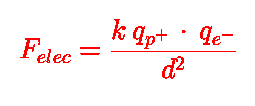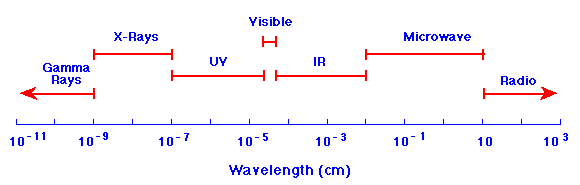# University of California, San Diego Center for Astrophysics & Space SciencesGene Smith's Astronomy Tutorial Newton's Laws, Basic Forces & Light### NEWTON'S LAWS OF MOTION

Here's another look at Newton's Laws.

### Newton's First Law -- Inertia

Unless a body is acted upon by an outside influence (force), it will remain in uniform motion. A body at rest will remain at rest; a moving body will continue moving at the same speed in the same direction.

Here is another look at Newton's First Law.

### F = ma

• Mass is the measure of the inertia of the body - how hard it is to get the body moving (grams).
• Force is the outside influence (dynes - like our Chancellor - in cgs units).
• Acceleration is the change in motion; this can be speeding up, slowing down, or changing direction (cm/s2)
Another look at Newton's Second Law.

### Newton's Third Law -- Action & Reaction

 When body A exerts a force on body B, body B exerts an equal and opposite force on body A. FA B = - F BA Often stated as the Law of Action and Reaction, the Third Law is responsible for Conservation of Momentum and is the Principle that makes Rocket Flight possible.Another look at Newton's Third Law.

As far as we know, there are four Fundamental Forces:Gravity Weak Nuclear Force Electromagnetism (or Electricity) The Strong Nuclear Force
in increasing order of strength. For another look at the fundamental forces follow this link.

### Gravity - The First Fundamental Force

Newton knew from his Third Law that the Force of Gravity must be reciprocal between the two masses involved. By applying his newly developed mathematical tool called fluctions (i.e. calculus), to the orbit of the Moon around the Earth, he was able to determine that the force must depend upon the inverse square of the distance between the Earth and the Moon. The gravitational force between the Earth and the Moon, or any two masses, is given bywhereis the mass of the Earth, andis the mass of the Moon, d is the distance between the Earth and the Moon, and G is Newton's Gravitational constant (G = 6.67 x 10-8dyne.cm2/g2) Gravity is such a weak force that Newton's constant could not be measured at that time. The first to estimate the value of G was astronomer Nevil Maskelyne. He used the deflection of a plumbline on the slopes of Mt. Schiehallion in Scotland to determine the gravitational attraction between the plumbob and the mountain in 1774. From Schiehallion's very regular shape, Maskelyne was able to estimate the mountain's mass and thus determine the value of G. Henry Cavendish was the first to measure G in the laboratory

Despite the fact that Gravity is the weakest of the fundamental forces, because it is a purely attractive, long-range force, we will see that Gravity dominates many areas of astronomy. These include the orbits of planets,stars, and galaxies, the life cycles of stars, and the evolution of the Universe.

The Electromagnetic Force

The electric force between electrons and protons is given bywhere q is electrical charge, d is distance and k is a constant for electricity (analogous to G for gravity). The form of the equation for electrical force is the same as that for gravity, but there are some key differences:

• There is only one kind of mass whereas there are two kinds of electrical charge - positive and negative - which behave in opposite fashion.
• Gravitation is purely attractive, but the electrical force can be either attractive or repulsive - like charges repel, opposite charges attract.+   +-   --+

• Moving charges produce and respond to the magnetic force. It turns out that the electric and magnetic forces are different manifestations of the same thing, due to Special Relativity. For this reason, astronomers & physicists speak of the "Electromagnetic Force".
Although the Electromagnetic force is of order 1039 times stronger than Gravity, it is not as apparent because of its dual (attractive/repulsive) nature. This causes most regions of space to be electrically neutral. The forces which prevent you from falling through the floor or your chair, the forces that are exerted when you push an object, (friction, etc.) are all examples of the electromagnetic force in action.

The Weak Force

The Weak Force is about 10-13 times as strong as the Electromagnetic force, with a range of only 10-16 centimeters. The Weak Force moderates certain kinds of nuclear decays such as the neutron decay shown below. The most common particle which interacts only via the Weak Force is the neutrino, symbol Greek "nu" below. We shall hear more about the neutrino when we talk about Nuclear Reactions & Stellar Evolution and Cosmology.We now know that the Weak Force is an aspect of a "unified force" called the "Electro-Weak Force" which combines the properties of the Weak Force and the Electromagnetic Force. Many physicists believe that all of the forces are really different aspects of a single "Grand Unified Force." Progress is being made toward combining the Strong Nuclear Force with the Electroweak Force, but as yet how to include Gravity remains a problem.

The Strong (Nuclear) Force
The Strong Nuclear Force (also called the "nuclear force" or the "strong force") holds the atomic nucleus together. To do this, it must overcome the tremendous repulsion that arises when the positively charged protons are packed together into the small space of the nucleus. While the nuclear force is the strongest of the fundamental forces, it has a very short range, effectively only the scale of the atomic nucleus ~ 10-13 cm. So, the strong force can only overcome the electrical repulsion when the protons are close enough to be "touching". Because neutrons interact with protons via the Strong Force, but have no charge, more and more neutrons are necessary to "dilute" the repulsive forces and hold heavy nuclei together.

For this part of the tutorial we will treat light in terms of its wave properties. (We will see in the tutorial on Atomic Structure that light also behaves as a "particle" called a photon).We characterize a wave by its:

• frequency (; - Greek "nu"): the number of wavecrests per second (units: Hz or 1/s).
• wavelength (- Greek "lambda"): distance between wavecrests (units: cm or Å Ångstroms = 10-8cm; you will also see nm = 10-9m = 10-7cm = 10Å)
• speed, c = 3 x 1010cm/s in vacuum.
Ifwavecrests pass a point each second, each separated bycm, then the speed must be:

speed = c =x= frequency x wavelength

The wave can also be characterized by its energy, E:

Energy = h .where h = 6.625 x 10-27erg-sec is Planck's Constant. For example KGB radio, 101.5 FM, has a frequency = 101.5 megahertz = 101,500,000 cycles/sec. This must correspond to a wavelength of about 295cm between peaks in the electric and magnetic fields of the wave. The energy of this radio photon is 6.7 x 10-19erg. Considering that an erg is about the energy that a fly expends taking off from a wall, it's apparent that radio waves don't carry much energy. (Considering the long wavelengths of radio waves, it also doesn't make much sense to talk about radio photons!)

Visible light, radio waves, microwaves, x-rays; they are all electromagnetic radiation. They each have a certain range of wavelengths and energies, and they represent a region in the Electromagnetic Spectrum:• Gamma-rays: Below 0.1Å, highest frequency, shortest wavelength, highest energy.
• X-Rays: 0.1Å -- 100Å
• Ultraviolet light: 100Å -- 3000Å
• Visible light: 3000Å -- 10000Å = 1µm (micrometer or micron)
•• Infrared Light: 1µm -- 1mm
• Radio waves: Above 1mm, lowest frequency, longest wavelength, lowest energy.

To understand more about Infrared Light see this excellent Infrared Tutorial from NASA's Infrared Processing & Analysis Center at Caltech. The Chandrasekhar X-Ray Observatory's X-Ray Field Guide will tell you what you need to know about x-rays.

### Atmospheric Transmission

Not all wavelengths of light are transmitted through the Earth's atmosphere (fortunately for us). The Earth's atmospheric windows are shown below.Transmission of the Earth's atmosphere; the white region indicates the altitudes where light from the different spectral region does not penetrate. From NASA's Imagine

Atoms and ions in the Earth's upper atmosphere absorb gamma-rays, x-rays, and ultraviolet light; the atmosphere is opaque to these three forms of radiation. (The UV which is responsible for sunburn and skin cancer is "very-violet" visible light by our astronomical definition.) The atmosphere is transparent to visible light. Molecules (H2O, CO2, CH4...) absorb most wavelengths of infrared light; there are windows at 1.25µm, 1.65µm, 2.0-2.5µm, 3-4µm, 4.5-5.5µm,8-12µm, and 17-25µm. Then the atmosphere is largely opaque out to millimeter wavelengths. The atmosphere is transparent through the Radio to meter wavelengths, where the ionisphere reflects radio waves.

Atmospheric Seeing The Earth's atmosphere has another negative effect on astronomical research. Turbulence in the Earth's atmosphere produces the twinkling of the stars which astronomers call seeing. Turbulent cells in the Earth's atmosphere act like small lenses, refracting light rays as they pass through and blurring an image. This site has a graphic demonstration of the effects of seeing on photographs, and here is an mpeg animation of Betelgeuse, from the 4-m Herschel Telescope in the Canary Islands,courtesy of the Applied Optics Group, Imperial College, London.The US at Night: Note the near solid patch of city lights from Los Angeles to San Diego